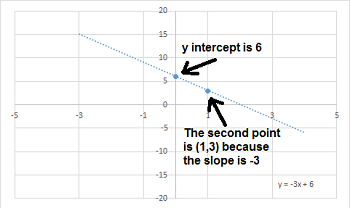# How to Solve Systems of Linear Equations by Graphing

Instructor: Betsy Chesnutt

Betsy teaches college physics, biology, and engineering and has a Ph.D. in Biomedical Engineering

Systems of linear equations are important in many branches of math and science, so knowing how to solve them is important. In this lesson, learn one way to find the solution of a system of linear equations by graphing.

## What is a System of Linear Equations?

In this lesson, you will learn how to solve a system of linear equations by graphing. Before you can do that, though, you need to know how to recognize a system of linear equations. First, a linear equation is one that forms a line when graphed. It has two variables, usually x and y, and typically looks like this:

y = 3x + 5

or maybe this:

6y + 3x = 9

A system of linear equations is made up of two linear equations. To solve the system of equations, you need to find the exact values of x and y that will solve both equations. One good way to do this is to graph each line and see where they intersect.

Before you can graph a linear equation, you need to make sure that it is written in slope-intercept form:

Slope-intercept form of a linear equation: y = mx + b

In slope-intercept form, m is the slope of the line and b is the y-intercept, so in the equation above, y = 3x + 5, the slope would be 3 and the y-intercept would be 5.

## How to Graph a Line using a Linear Equation

Now that we know how to recognize a linear equation, let's review how to graph a line. First, you want to rearrange the equation so it's in slope-intercept form. Let's see how to do that with this equation:

3y + 9x = 18

First subtract 9x from both sides:

3y = -9x + 18

Then divide both sides by 3:

y = -3x + 6

Now, you can tell that the slope of the line (m) is -3 and the y intercept (b) is 6. To graph this line, you can use a graphing calculator or computer, but you can also do it by hand on paper. First, the y-intercept is the point where the line crosses the y axis, so you can plot this point first. Then, look at the slope. The slope is a ratio of how far the line goes up in the y direction divided by how far it goes over in the x direction.

slope = change in y / change in x

So, a slope of -3 means that you should go down 3 units in the y direction for every 1 unit you go over in the x direction. You can use that to plot a second point and then use a ruler to connect the points and make a straight line.## How to Solve a System of Linear Equations by Graphing

To solve a system of linear equations by graphing, you will graph both lines and then see where they intersect each other. The x and y coordinates of the intersection will be the solution to the system of equations!

Why is this intersection point the solution to the system of equations? This is the only point that falls on both lines so it's the only combination of x and y values that will make each equation true.

Let's look at an example. Here are two linear equations that form a system of equations:

y = -3x + 6

y = 2x + 16

Graph both of these lines and then see where they intersect each other.

We already saw above that for the first equation, -3 is the slope while the y-intercept is 6.

To unlock this lesson you must be a Study.com Member.

### Register to view this lesson

Are you a student or a teacher?

### Unlock Your Education

#### See for yourself why 30 million people use Study.com

##### Become a Study.com member and start learning now.
Back
What teachers are saying about Study.com

### Earning College Credit

Did you know… We have over 160 college courses that prepare you to earn credit by exam that is accepted by over 1,500 colleges and universities. You can test out of the first two years of college and save thousands off your degree. Anyone can earn credit-by-exam regardless of age or education level.